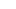# FREE Printable Aquarium Subtraction Math Games for Grade 1

Make math fun for first graders with this super cute subtraction games for grade 1. Children will solve the subtraction equations on the cute fish and then put them in the aquarium with the correct difference. This printable subtraction games is fun for first grade and  grade 2 students. Simply print pdf file with 1st grade subtraction game and you are ready for a fun math file folder games.## Subtraction games

This Aquarium Subtraction Game is a fun way to help 1st and 2nd grade students practice subtraction with a fun math game! This subtraction games for grade 1 is a math file folder games which is a fun, easy-to-store, engaging subtraction activity for kids. Use this subtraction activity as a math center, enrichment, summer learning, and extra subtraction practice to help work on math fluency.

## Math Aquarium

Start by scrolling to the bottom of the post, under the terms of use, and click on the text link that says >> _____ <<. The fish printables pdf file will open in a new window for you to save the freebie and print the template.## Subtraction games for grade 1

To make the file folder game. Print on cardstock. Attach the front of the cover and tab label as seen above. To make the aquarium pockets follow these directions:## Subtraction game

You can laminate the fish equations for durability & cut out. The complete assembly of this file folder game should take less than 30 minutes! I like to color code mine by grade for ease of use## Aquarium Math

Now you are ready to let your kiddo have at the fun and practice math! Simply have children solve the subtraction to 20 equation on one of the colorful fish. When children find the difference, have them put the fish into the correct aquarium featuring the numeral they solved for. When all the fish are happily swimming in their tank – you’re done!

This is better than subtraction worksheets because students can practice over and over again to achieve math fluency!

## Subtraction Activities

Looking for more subtraction worksheets and subtraction games to make learning to subtract fun? Check out these subtraction math ideas and free resources:

## Subtraction Printables

Have other children you are homeschooling? You will want to check out our pre k worksheets, free printable worksheets for kindergarten, grade 1 worksheets, grade 2 worksheetsand free alphabet printables. Plus find our history for kids and easy science experiments for kids or all our free printable worksheets for kids.

## Subtraction Activity

By using the printable below you agree to the following:

• This is for personal use only (you may print off 1 copy for your families personal use)
• This may NOT be sold, hosted, reproduced, copied or stored on any other site (including blog, Facebook, Dropbox, etc.)